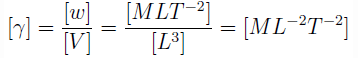# Test: Fluid Properties - 1

## 10 Questions MCQ Test Fluid Mechanics | Test: Fluid Properties - 1

Description
Attempt Test: Fluid Properties - 1 | 10 questions in 20 minutes | Mock test for Mechanical Engineering preparation | Free important questions MCQ to study Fluid Mechanics for Mechanical Engineering Exam | Download free PDF with solutions
QUESTION: 1

### Which one of the following is the unit of specific weight?

Solution:

Explanation: Specific weight(γ) is defined as the weight(w) per unit volume(V ), i.e.,
γ = w / v
Thus, unit of is N / m3.

QUESTION: 2

### The specific gravity of a liquid has

Solution:

Explanation: The specific gravity of a liquid is the ratio of two similar quantities (densities) which makes it unitless.

QUESTION: 3

### The specific volume of a liquid is the reciprocal of

Solution:

Explanation: Specific volume(v) is defined as the volume(V ) per unit mass(m).
v = v⁄m = 1 / m⁄v = 1⁄p
where p is the mass density.

QUESTION: 4

Match the following physical quantities in Group 1 with their dimensions in Group 2.

1. Mass Density (p)                                                 A. [M0 L 0 ]
2. Specific Gravity                                                  B. [M L– 1 T – 1 ]
3. Specific Volume (v)                                            C. [ML -3 0 ]
4. Specific Weight (γ)                                             D. [M-1 L3]
5. Dynamic viscosity (μ)                                        E. [M L– 2 – 2 ]

Solution:

Explanation:

-Mass Density(p) is defined as the mass(m) per unit volume(V ), i.e.,
[p] = [m]/[v] = [m] /[L3] = [ML-3]

-The specific gravity of a liquid is the ratio of two similar quantities (densities) which makes it dimensionless.

-Specific volume(v) is defined as the volume(V ) per unit mass(m). Thus,
[v] = [V]/[m] = [L3]/[M] = [M-1L3].

-Specific weight(γ) is defined as the weight(w) per unit volume(V ), i.e.,QUESTION: 5

Which of the following forces generally act on fluid while considering fluid dynamics?

1. Viscous force
2. Pressure force
3. Gravity force
4. Turbulent force
5. Compressibility force

Solution:
QUESTION: 6

Which property of the fluid offers resistance to deformation under the action of shear force?

Solution:
QUESTION: 7

The study of force which produces motion in a fluid is called as

Solution:

Explanation: Fluid dynamics is the study of forces responsible for the motion of fluids.

QUESTION: 8

The specific weight of the fluid depends upon

Solution:
QUESTION: 9

Two fluids 1 and 2 have mass densities of p1 and p2 respectively. If p1 > p2, which one of the following expressions will represent the relation between their specific volumes v1 and v2?

Solution:

Explanation: Specific volume(v) is defined as the volume(V ) per unit mass(m).
v = v⁄m = 1 / m⁄v = 1⁄p
where p is the mass density. Thus, if p1 > p2, the relation between the specific volumes v1 and v2
will be represented by v1 < v2.

QUESTION: 10

A beaker is filled with a liquid up to the mark of one litre and weighed. The weight of the liquid is found to be 6.5 N. The specific weight of the liquid will be

Solution:

Explanation: Specific weight(γ) is defined as the weight(w) per unit volume(V ), i.e.,
γ = w⁄V
Thus, γ = 6.5 ⁄10-3 N ⁄ m3 = 6.5 kN/m3.Use Code STAYHOME200 and get INR 200 additional OFF Use Coupon Code